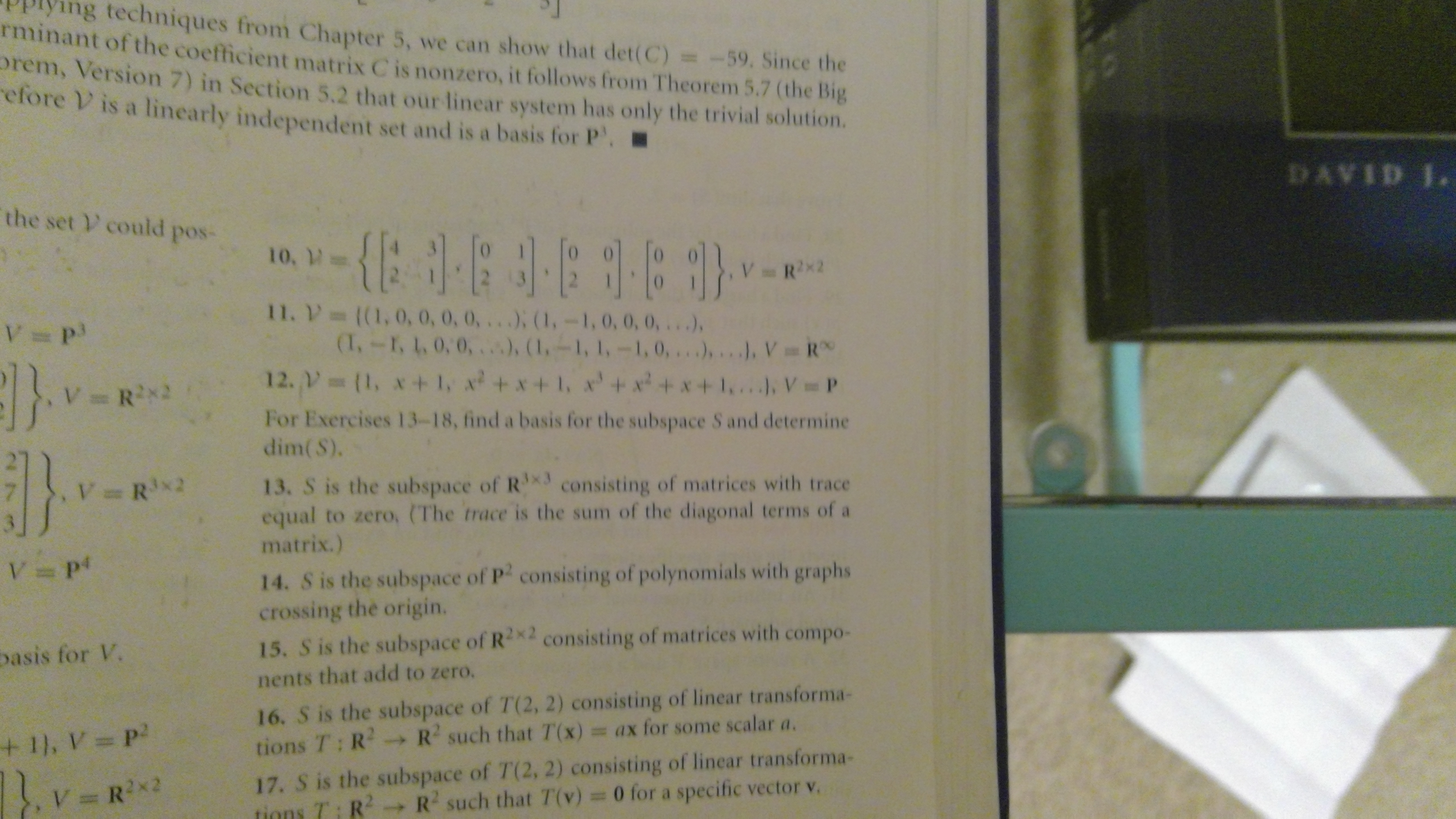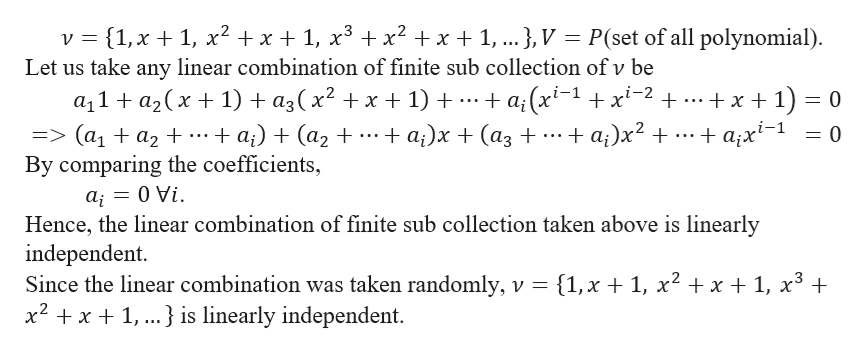# ing techniques from Chapter 5, we can show that det(C) = -59. Since theminant of the coefficient matrix C is nonzero, it follows from Theorem 5.7 (the Bigrem, Version 7) in Section 5.2 that our linear system has only the trivial solution.efore V is a linearly independent set and is a basis for P.DAVID Ithe set V could pos-10,VRx211. V- ((1,0, 0, 0, 0, ...), (1,-1, 0, 0, 0, ...)(1,-1, 1, 0,0,.), (1,-1, 1,-1,0,...),. ., VR12. (1, x+ 1, x+x+1, x+ x2+ x+ 1. ). V=PV PVR2For Exercises 13-18, find a basis for the subspace S and determinedim(S).13. S is the subspace of R consisting of matrices with traceequal to zero, (The trace is the sum of the diagonal terms of amatrix.)VRx214. S is the subspace of P2 consisting of polynomials with graphscrossing the origin.15. S is the subspace of R2x2 consisting of matrices with compo-basis for V.nents that add to zero.16. S is the subspace of T(2, 2) consisting of linear transforma-tions T: R R2 such that T (x) = ax for some scalar a.+ 1, V= P17. S is the subspace of T(2, 2) consisting of linear transforma-tions T: RVRx2R such that T(v) = 0 for a specific vector v.

Question
40 views

For Problem #12, how do I prove that the set is a basis for V? I think that infinity is the basis, but I'm not sure. This is a Linear Algebra type of question. Here is a picture.help_outlineImage Transcriptioncloseing techniques from Chapter 5, we can show that det(C) = -59. Since the minant of the coefficient matrix C is nonzero, it follows from Theorem 5.7 (the Big rem, Version 7) in Section 5.2 that our linear system has only the trivial solution. efore V is a linearly independent set and is a basis for P. DAVID I the set V could pos- 10, VRx2 11. V- ((1,0, 0, 0, 0, ...), (1,-1, 0, 0, 0, ...) (1,-1, 1, 0,0,.), (1,-1, 1,-1,0,...),. ., VR 12. (1, x+ 1, x+x+1, x+ x2+ x+ 1. ). V=P V P VR2 For Exercises 13-18, find a basis for the subspace S and determine dim(S). 13. S is the subspace of R consisting of matrices with trace equal to zero, (The trace is the sum of the diagonal terms of a matrix.) VRx2 14. S is the subspace of P2 consisting of polynomials with graphs crossing the origin. 15. S is the subspace of R2x2 consisting of matrices with compo- basis for V. nents that add to zero. 16. S is the subspace of T(2, 2) consisting of linear transforma- tions T: R R2 such that T (x) = ax for some scalar a. + 1, V= P 17. S is the subspace of T(2, 2) consisting of linear transforma- tions T: R VRx2 R such that T(v) = 0 for a specific vector v. fullscreen
check_circle

Step 1

We are giv...help_outlineImage TranscriptioncloseP(set of all polynomial) v 1, x1, x2 x1, x3 x2 x1, ... }, V Let us take any linear combination of finite sub collection ofv be a;(x1x2 .. +x 1) 0 + аx*-1 a11a2x 1) + a3(x2 + x + 1) 3> (ај + аz t+ By comparing the coefficients, +а) + (аz + + a;)х + (аз + = 0 Vi а; Hence, the linear combination of finite sub collection taken above is linearly independent. Since the linear combination was taken randomly, v {1,x + 1, x2 + x + 1, x3 x2 x1,..} is linearly independent fullscreen

### Want to see the full answer?

See Solution

#### Want to see this answer and more?

Solutions are written by subject experts who are available 24/7. Questions are typically answered within 1 hour.*

See Solution
*Response times may vary by subject and question.
Tagged in

### Math